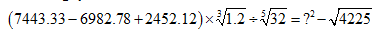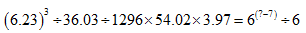# Approximation Quiz With Solutions: Part 91. What approximation value should come at the place of question mark (?) in the following question?

A. 196
B. -196
C. -14
D. 16
E. -16
Ans. C.

2. What approximation value should come at the place of question mark (?) in the following question?

A. 3025
B. 4225
C. 1225
D. 2025
E. 6250

3. What approximation value should come at the place of question mark (?) in the following question?A. 41
B. 43
C. 49
D. 59
E. 39

4. What approximation value should come at the place of question mark (?) in the following question?A. 8
B. 5
C. 7
D. 9
E. 4

5. What approximation value should come at the place of question mark (?) in the following question?A. 1740
B. 1340
C. 1250
D. 1470
E. 1860

#### 5. B. 1340#### What's trending in BankExamsToday

Smart Prep Kit for Banking Exams by Ramandeep Singh - Download here